Processing ......FreeComputerBooks.com Links to Free Computer, Mathematics, Technical Books all over the World

Computer Algebra in Scientific Computing
🌠 Top Free Unix/Linux Books - 100% Free or Open Source!
• Title Computer Algebra in Scientific Computing
• Author(s) Andreas Weber, et al.
• Publisher: Mdpi AG (November 4, 2019); eBook (Internet Edition)
• Permission: Link to the Internet Edition of the book in publisher's homepage.
• Hardcover/Paperback 160 pages
• eBook PDF Files
• Language: English
• ISBN-10/ASIN: 3039217305
• ISBN-13: 978-3039217304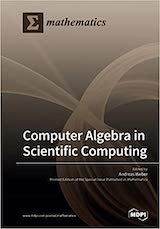Book Description

Although scientific computing is very often associated with numeric computations, the use of computer algebra methods in scientific computing has obtained considerable attention in the last two decades. Computer algebra methods are especially suitable for parametric analysis of the key properties of systems arising in scientific computing. The expression-based computational answers generally provided by these methods are very appealing as they directly relate properties to parameters and speed up testing and tuning of mathematical models through all their possible behaviors.

This book contains 8 original research articles dealing with a broad range of topics, ranging from algorithms, data structures, and implementation techniques for high-performance sparse multivariate polynomial arithmetic over the integers and rational numbers over methods for certifying the isolated zeros of polynomial systems to computer algebra problems in quantum computing.

• Katta Gopalakrishna Murty is a Professor, Industrial and Operations engineering, The University of Michigan, Ann Arbor.
Reviews, Ratings, and Recommendations: Related Book Categories: Read and Download Links:Similar Books:
•Tea Time Numerical Analysis: Experiences in Mathematics

This textbook was born of a desire to contribute a viable, free, introductory Numerical Analysis textbook. The ultimate goal of this book is to be a complete, one-semester, single-pdf, downloadable textbook designed for mathematics classes.

•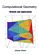Computational Geometry: Methods and Applications (Jianer Chen)

An introduction to some of the fundamental principles of computational geometry, concentrates on four major directions in computational geometry: the construction of convex hulls, proximity problems, searching problems and intersection problems.

•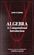Algebra: A Computational Introduction (John Scherk)

This book is a unique approach and presentation, the author demonstrates how software can be used as a problem-solving tool for algebra. It includes many computations, both as examples and as exercises.

•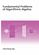Fundamental Problems of Algorithmic Algebra (Chee-Keng Yap)

This book provides a systematic and focused treatment of a collection of core problemsthe computational equivalents of the classical Fundamental Problem of Algebra and its derivatives.

•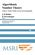Algorithmic Number Theory: Lattices, Curves, and Cryptography

This text provides a comprehensive introduction to algorithmic number theory for beginning graduate students, written by the leading experts in the field.

•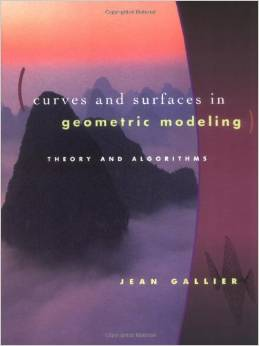Curves and Surfaces in Geometric Modeling: Theory and Algorithms

This book offers both a theoretically unifying understanding of polynomial curves and surfaces and an effective approach to implementation. It is also an exellent introduction to geometry concepts used in computer graphics, vision, robotics, geometric modeling.

•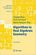Algorithms in Real Algebraic Geometry (Saugata Basu, et al)

The monograph gives a self-contained detailed exposition of the algorithmic real algebraic geometry. It will be useful both for beginners and for advanced readers, who work in real algebraic geometry or apply its methods in other fields.

•Algorithmic Algebra (Bhubaneswar Mishra)

Anyone need to use algorithms in polynomials should have a copy of this! This is definitely one of the best! It covers almost everything anyone need in computation with systems of polynomial equations: Grobner Basis, Resultants, Characteristic Set, etc.

•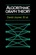Algorithmic Graph Theory (David Joyner, et al)

This is an introductory book on algorithmic graph theory. Theory and algorithms are illustrated using the Sage open source mathematics software. It's especially suitable for computer scientists and mathematicians interested in computational complexity.

•Applied and Computational Linear Algebra: A First Course

This book is intended as a text for a graduate course that focuses on applications of linear algebra and on the algorithms used to solve the problems that arise in those applications.

Book Categories
 :All CategoriesTop Free BooksRecent BooksMiscellaneous BooksComputer EngineeringComputer LanguagesComputer ScienceData Science/DatabasesJava and Java EE (J2EE)Linux and UnixMathematicsMicrosoft and .NETMobile ComputingNetworking and CommunicationsSoftware EngineeringSpecial TopicsWeb Programming
Other Categories